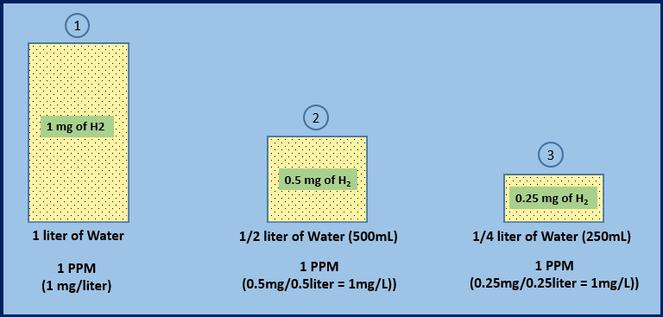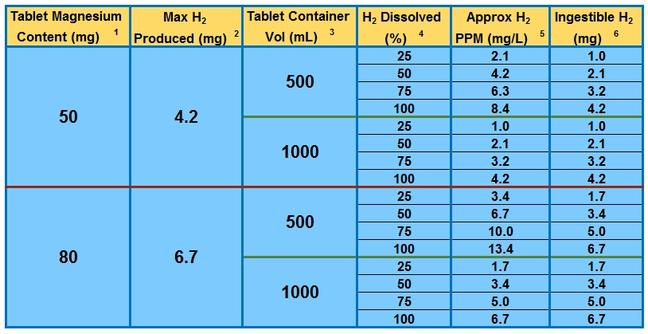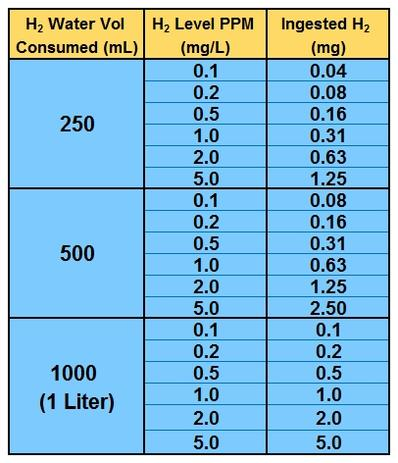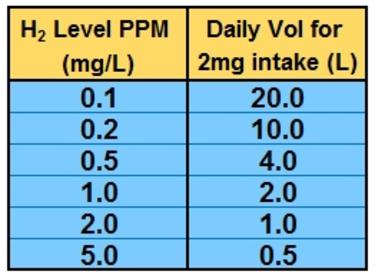## What is PPM?

PPM is an acronym for "Parts Per Million, a method of measuring how much of one substance (called the "solute") is dissolved in water or another liquid (called the "solvent"). PPM's may also be expressed in an equivalent unit of measure, mg/L (milligrams per liter). Converting between these two units is simple: 1PPM = 1mg/L

## Why Is PPM the Same as mg/L?

As stated above, 1PPM = 1mg/L, but why is this true?
Here is a detailed explanation: We can think of 1PPM as "1 part of a substance (solute) dissolved in 1 million parts of water" (in our case the solute is hydrogen gas).
So, what is a "part"?
"Part" represents our unit of measure, the milligram (mg). Therefore, 1 milligram of H2 (1 part) dissolved in 1 million milligrams of water is "one part per million". And, because 1 liter of water weighs 1 million milligrams, the mg/L unit of measure can be equated to the PPM (for dilute concentrations).

## What does the PPM Reading Tell Me about my Hydrogen Water?

When using H2Blue to measure a sample of hydrogen water, each drop represents 0.1 PPM of dissolved H2 gas. By adding together the total number of drops required to reach the titration endpoint, the dissolved H2 level in PPM can be measured (see TECHNICAL page). Because the results of each measurement done with H2Blue are expressed in PPM (or mg/L), it is important to understand what the PPM units mean. In other words, "how much hydrogen will I ingest if I drink the entire container?". How the results are interpreted depends upon how the hydrogen water was originally produced.​

## Hydrogen Water Produced using Hydrogen Tablets:

Hydrogen tablets produce hydrogen gas through the reaction of elemental magnesium (Mg) and water:

​ Mg + 2H2O => Mg(OH)2 + H2

The production of the gas bubbles can be easily observed when placing a tablet in a clear container, and in a closed container, the pressure will rise as the volume of H2 gas produced increases.

Distinguishing Between "Produced" and "Dissolved" Hydrogen Gas:

Because each tablet contains a fixed amount of magnesium metal, usually in the range of 50 to 80 milligrams, the total amount of H2 gas which can be produced from each tablet is also fixed. Although the theoretical maximum amount of H2 gas which can be produced from one tablet (under ideal conditions) can be calculated based upon the amount of magnesium in the tablet (usually indicated on the label), not all of the H2 gas produced will actually dissolve into the water. This is because a portion of the H2 gas will escape into the air and be wasted. The percentage of H2 produced which actually dissolves into the water is typically in the 25% to 50% range. This percentage can be influenced by factors such as temperature, time & pressure. Only the dissolved H2 gas can be considered when calculating ingestible H2 levels.

Converting the Amount of Ingestible H2 based on PPM Reading and Container Size:

In order to calculate the amount of ingestible dissolved H2 present in a given container using the H2Blue PPM reading, the size of the container into which the tablet was placed (and from which the 6ml test sample is taken) must be taken into consideration. This is because it takes fewer milligrams of H2 to produce 1PPM in smaller amounts of water than it does in 1 liter of water. The following graphic illustrates this concept:​In this example, a 6ml sample taken from each container will measure the same, 1PPM (10 drops of H2Blue). But, these containers DO NOT contain the same AMOUNT of dissolved H2. As the volume of water is halved, so too is the amount of dissolved H2, resulting in the SAME concentration in each container. From this example, it can be seen that PPM's DO NOT tell the entire story about how much H2 will be ingested when drinking the H2 water being sampled. We must relate the test sample to the size of the container from which it was taken to know how much H2 will be ingested when the water is consumed.

​​​Calculating the Theoretical Maximum Amounts of H2 which can be Produced and Dissolved:

Utilizing the number of milligrams of Magnesium contained in the tablet, we can:

• Calculate the theoretical maximum amount of H2 which can be produced

From which we can then also:

• Approximate the maximum level of dissolved H2 (PPM) and ingestible H2 (mg) which can be expected.

When calculating ingestible H2 levels, it is important to remember that only DISSOLVED H2 can be considered (regardless of how much H2 is produced). A few variables come into play which have an impact on the percentage of H2 gas which ultimately dissolves into the water. For any given tablet size, these variables include:

• ​Whether or not the container can maintain pressure - pressure helps H2 dissolve better.
• Materials from which the container is constructed - less-porous materials hold H2 better.
• The amount of time the tablet is permitted to dissolve - more time (hours) is generally better than less time (minutes)
• The temperature of the water - H2 will dissolve better in cold water
While a larger container will produce lower PPM readings, the amount of ingestible H2 will not necessarily be less.

Table 1 below shows the maximum H2 production possible, and the levels of measured PPM and ingestible H2 one can expect based on some tablet sizes, container volumes, and dissolved H2 percentages:Table 1

How to use this table:
• Select the value in column 1 corresponding to the amount of magnesium in your tablet, usually found on the label (if using two tablets, double the results shown in columns 2,5 & 6)
• Column 2 shows the maximum amount of hydrogen gas which can be produced from the tablet (under ideal conditions), based on the reaction stoichiometry and molecular weights.
• Select the value in column 3 corresponding to the volume of water into which the tablet is to be dissolved.
• Select the value in column 4 corresponding to the anticipated percentage of dissolved hydrogen. Values will vary depending on preparation method, but will typically be in the 20% to 50% range.
• Column 5 will give the approximate PPM which you would expect to measure based upon the amount of magnesium in the tablet, container volume, and % of the produced H2 which dissolves.
• Column 6 will give the approximate amount of H2 which will be ingested if one drinks the contents of the container.
Example: One 50mg tablet is capable of producing 4.2mg of H2. If it is placed into a 500mL container, allowed to dissolve for 20 minutes, and we assume that 25% of the H2 which can be produced will dissolve, then a concentration of approximately 2.1PPM will be measured, and approximately 1mg of H2 will be ingested when consuming the entire contents.​

## ​Hydrogen Water Produced using Electrolytic Devices such as Alkaline Ionizers and Hydrogen Infusion

Alkaline ionizers produce hydrogen water by reducing (adding electrons) protons (H+) to H2 at the cathode: ​

2H+ + 2e- => 2H2

Hydrogen gas then dissolves into the drinking water as the water flows across the cathode.

Recently, a new type of hydrogen water device, the hydrogen infusion machine (HIM), has emerged, which produces hydrogen gas in a separate chamber using a proton-exchange membrane (PEM), and then mixes the H2 gas into the drinking water stream using a dissolver. Because of their design, HIM's do not alter the pH of the hydrogen drinking water like conventional alkaline water ionizers.

PPM's and the Difference Between Tablets and Electric Devices:

While a hydrogen tablet contains a "fixed" amount of magnesium, the source of electrons for producing H2 gas, electric devices have no such limitation, and therefore have an "infinite" supply of electrons for producing H2 gas (as long as there is power available). As a consequence, we need not be concerned with the container size from which the 6mL test sample is taken when interpreting the PPM's measured using H2Blue. We know that, since electric devices can produce H2 water continuously, any sample taken from the machine will be representative of its ability to provide any volume of H2 water (within the design constraints of the machine). Therefore, to know how much H2 we will ingest, we only need to know two things:

1) The H2 concentration (in PPM) of the water it produces

2) The volume of water consumed.

The following table shows ingested H2 levels for consumption volumes at various PPM concentrations:​Table 2

## How Much H2 Do I Need?

Now that we understand PPM's and how to interpret them to tell us the amount of dissolved hydrogen gas contained within a particular volume, the question becomes

"How much H2 do I need to ingest everyday"?

Scientists are asking the same question, and the answer is currently under investigation. What we do know is that human studies show significant health benefits when participants consume hydrogen water with concentrations in the 1-3 mg/L range. This gives us a "target" dosage of approximately 2 mg/day. Therefore, if your water has a concentration of 1 PPM (1 mg/L), then by consuming two liters per day, you will ingest 2 mg of H2 per day. Based upon the H2 concentration of your water, you can use Table 3 below to calculate the daily volume of water required to provide 2 mg of H2.Table 3

From Table 3 it can be seen that as the dissolved hydrogen concentration falls below 0.5 PPM, the amount of water which must be consumed to deliver 2 mg/day of H2 becomes prohibitively high. Therefore, it is important to choose a device/technology which is capable of delivering H2 water at or above 1 PPM.